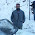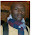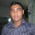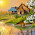## Friday, January 14, 2011

### Generation and Implementation of Sine Wave TableFor zooming:1.Tehmid,
Great and wish much more to come.

2.Tahmid i need your help with pwm in atmega16 my mail is aca.zamber@gmail.com

3.4.Dear Tahmid Great article, rare human without expecting any benefit from the society. I wish u all success.

5.Instead of the software "Sine Wave", I now use the software "Smart Sine", which you can find here:

http://tahmidmc.blogspot.com/2012/10/smart-sine-software-to-generate-sine.html

1.This comment has been removed by the author.

2.hello sir. i have been through your article related to SPWM and it is working fine and thanks for this. But my next point is i want to change frequency as well as voltage using a potentiometer. Please reply me

6.Owsum great work ..
i want to develope it using attiny13 .. will u help me in this ?? thanking u :)

7.Ok.

If you've understood how it works, you can make it using any microcontroller. As the ATTINY13 does not have any ECCP or similar module, you need to apply the SPWM technique a little differently to generate the required outputs.

8.Yeah but how?
m new in microcontrollers .. will u plz guide me ?

9.Use the PWM module to generate the required SPWM signals. Then you can use 2 or 4 external pins and additional hardware to drive that same SPWM output to 2 or 4 MOSFETs.

So your first task will be to generate the SPWM signals by first creating a sine table and then using that sine table to generate corresponding PWM signals.

10.in a three-phase inverter bridge six switches. how to get the pulse train for one as create table sine of 90 ° with 19 points?. then to form the three AC waveforms
I see, from 90 to 180 repeats of the 0-90 but in mirror, and then 180 to 360 would only be the opposite of the first half cycle, but when the three branches desfaso 120 and take the voltage between them do not see a way AC waveform.
(in this simulation). I will use the PIC18F4431 to implement the system. You can help.
also try to simulate with proteus AN900 microchip but only see PWM1-PWM3.
PWM3-PWM5 And PWM5-PWM1 is not there seems to be a problem with PWM5, does not change the frequency.

11.kindly give the simulation file

12.Hello Tahmid
Tour post on sine wave generator for dc-ac Sine wave Inverter was very helpful Please, Can you help with a tutorial on how to design and select a filler Inductor Core for filtering of the Sod wave Component from the spwm output . This has been a problem for me for quiet a while now. I will be very grateful for your assistance.
RegardS
Bello

13.can u plz specify numberz for FETs and Q1......Q4
and bro IR2110 is not available in protious?????????

14.Sir

With the given example you give 50Hz is we send 16x per sample, it mean for 60Hz we have to send it 13x, am I right please, please advise.

regards,

15.اسلام علیکم
Tahmid weldone very useful work ...

16.Thanks for sharing, very usefull.

17.can you please help me to code in ccs c and then using that 16Khz as carrier and then make a dc-ac converter ?

Thanks.

1.I don't use CCS C; so I can't really help on that regard.

Look at all the articles under "SPWM and sine wave generation and inverter".

If you need help porting to CCS, feel free to ask and I'll try to explain the corresponding mikroC statements.

Regards,
Tahmid.

2.sir, i found this article very help full i am doing FYP on SMPS.
sir, I will ask you for help in this regard.
Thanks

18.Thanks for the support given to the implementation of the sine wave table.
Question: Do you multiply the values ​​by 250 V peak breasts to get the final values ​​of the table. In my country the voltage is 220 Vrms, I would multiply the values ​​breasts (220x1.414)? . Thanks for the reply.

1.I'm not quite clear regarding your question. Could you clarify?

19.Hi Tahmid:
I repeat my question. Once you have calculated the 32 values ​​of the sine wave:
sin (0) = 0
sine (18) = 0.31
----
----
sine (162) = 0.31

Because multiply by 250, this value (250) for this case corresponds to the peak voltage is to be output sine wave?

Thanks for all the support.

1.No, that's not for the peak voltage of the output sine wave. That's for the peak voltage of the PR2 register. It doesn't matter what your peak sine wave voltage is. That multiplication factor (250 here) is set by the value of the PR2 register and the peak value you want the sine wave table to have (usually equal to PR2).

Regards,
Tahmid.

20.hi Tahmid,
I want u help plz send u email address;

21.Hi Tahmid:
To clarify my concept as the correction that do not match the frequency, not quite understand, because it is not what happens with the RC2 pin state in the loop of 16 repetitions. So I wrote the following to me confirmnes if correct.

When you start to develop the sine table, say take 10 parts for 50Hz half sine wave.
Then right thing would be to calculate the PWM period according to these data:
1/50 = 0.02 = 20.000 us.

Then each part is worth 20,000 / 20 = 1,000 us and this value would be the PWM period. This value would be used in the formula:
(PR2 +1) = PWM Period / (4x Tosc x TMR2 prescaler value)

Replacing values:
PWM period = 1000us
Tosc = 1/16, 000,000 = 0.625 us
TMR2 prescaler = 16

(PR2 +1) = 1000 / (4x 625 x 16)

PR2 = 24

Another Question: Here's another solution, TMR2 prescaler value = 4 thus PR2 = 99. The two numbers are integers, what is the best option to use a large value in PR2 or a small value.

Thanks for all the support.

22.Sorry Tahmid:
To complete my question and I'm clear all the following is correct?
If you say use a 16Khz carrier frequency, the period of this signal is 1/16, 000 = 62.5 us corresponding to PWM period.
With this data we calculate how many pieces we will divide 50Hz half sine wave. For this we proceed as follows:
1/50hz = 20,000 us
20,000 us/62.5us = 320 parts into which 50Hz sine wave.
Then half-wave 50Hz sine there are 160 parties and this data is constructed sine table.

Thanks

1.Yes, you've got that right.

One clarification though: all those 160 parts don't need to be in the table. You can have a lower number and just call that multiple times.

For example, I use 32 values in the sine table and call each one 5 times. So, in my half cycle, in the end, I end up having 32*5 = 160 values. That's what I need.

The advantage here is that you need a smaller sine table which saves a lot of ROM space.

Regards,
Tahmid.

2.So, am I right in concluding that the number "32" comes from "a fraction of comparison between carrier freq vs mains freq"?
i.e if carrier freq is 6kHz and mains freq is 50Hz, then the comparison value is 120 for full cycle, or 60 for the half cycle. then we can use any fraction of that 60 for the sine table size, maybe 20,30,or 60?

3.Yup, you've got it right.

23.How to make 50hz pwm(2 output) with feedback in picbasic pro?

1.Unfortunately, I don't think I can help you much with that since I have not used PICBASIC PRO. I could help you with the algorithm, but I can't help you with converting that to PICBASIC PRO code. You'll need to do that.

Regards,
Tahmid.

2.welll....
plz tell me the main ideas about PWM and include the C program.....

24.hei bro can u mail me ??? i m from aiub eee intrested about ur project my mail nahideeeaiub@gmail.com me in fb https://www.facebook.com/EEEnahid please give ur contac mail/cellno in my mail or fb id

25.Hi tahmid,
i designed an inverter which is transformer less.
Power =10KVA
PWM Generated Using Analog Circuit.
Topology =Half Bridge
Switching Frequency =10KHz
Currently i have connected RC snubber across both MOSFET & using same Bus Link Capacitor as filter capacitor also.
The Driver used can Sink & Source 4A current.
But i want to use anti-parallel diode across gate resistor,for fast turn off.Which Diode to choose as frequency is 10KHz?
Also snubber required is across both or single in half bridge?

Also Rg & Rgs resistor are connected.

Thanks & waiting for knowledge enhance,

26.Hi Tahmid
Your work is good and i think, now I am able to complete my project with in time

27.This comment has been removed by the author.

28.This comment has been removed by the author.

29.I want to make a pure sinewave inverter by using arduino.can you please guide for coding part.
The h bridge upper half are p-ch and lower half are n ch mosfet.

30.Please can you explain in detail the advantages of FULL BRIDGE over CENTER TAPPED inverters apart from extra coil cost. Lets assume a transformer is wound with 12SWG 25 turns at the primary for FULL BRIDGE, and the same size of transformer is used to wind 25-0-25 CENTER TAPPED with also 12SWG, what will be the difference if all other parts of the inverter is built with equal material.

31.Hello, this helped me alot. i just wanted to know that if i wanted to generate a 40Hz sine wave what changes do i have to make in the code?

32.HI Tahmid
I have already done an inverter single phase in the dsPIC BASIC PRO compiler using a dsPIC30F4012. It works ok.
My question is how to generate a 3 phase inverter. What are the issues to solve? What should be added?
Could you make please a tutorial to make a three phase inverter? may be using a dsPIC, provided it has the hardware (Motor Control PWM) that supports 6 outputs, for a full bridge 3 phase inverter.
I hope you can spare a little time to help me and a lot of other guys interested.

On the other hand, could you please recommend a book or pdf document to consult this issue?
Thanks a lot for your valuable help.

Alejandro Marquez
email: amarkues@hotmail.com

33.Thank you so much for taking your time to write about these things. This is really helpful. I have just found part of a solution for my project. thank you a lot Tahmid.

34.Hi tahmid I have got my softawere woking correctly in pic 16f690, but now i want to know the type of mosfets and diodes that can be used in this application

35.Good morning Tahmid Mahbub >
at first i want to thank you about your exertion and Information that you give us .
seconds i want to Definition for myself ^^ I'm rashad shubita ,16 year old from palestine and i hope to be your friends.
at end i understand your presentation after i read it 5 or 6 time , and i'm make my code different ^^ and this is the way i write my code ,please tell me if i make some mistakes.
let's start : F=1/T >>T=1/50=0.02*1000000=20000us So each part is worth 20000/400=50us this is pwm period >and i'm used 20mhz osc
and used 20Khz carrier frequency so 1/20000= 0.00005*1000000=50 this is Required ^^ now let's calculate PR2 >
0.00005=(PR2+1)*4*1/20000000*1>> 0.00005=X*0.0000002>> X=0.00005/0.0000002=250 >>>PR2=249 .
this is the code :
const unsigned char sin_table={0, 4, 8, 12, 16, 20, 24, 27, 31, 35, 39, 43, 47, 51, 55, 58, 62, 66, 70, 74, 77, 81, 85, 88, 92, 96,
99, 103, 106, 110, 113, 117, 120, 124, 127, 131, 134, 137, 141, 144, 147, 150, 153, 156, 159, 162, 165, 168, 171, 174, 177, 180,
182, 185, 188, 190, 193, 195, 198, 200, 202, 205, 207, 209, 211, 213, 215, 217, 219, 221, 223, 225, 226, 228, 229, 231, 232, 234,
235, 237, 238, 239, 240, 241, 242, 243, 244, 245, 246, 246, 247, 248, 248, 248, 249, 249, 250, 250, 250, 250, 250, 250, 250, 250,
250, 249, 249, 248, 248, 248, 247, 246, 246, 245, 244, 243, 242, 241, 240, 239, 238, 237, 235, 234, 232, 231, 229, 228, 226, 225,
223, 221, 219, 217, 215, 213, 211, 209, 207, 205, 202, 200, 198, 195, 193, 190, 188, 185, 182, 180, 177, 174, 171, 168, 165,
162, 159, 156, 153, 150, 147, 144, 141, 137, 134, 131, 127, 124, 120, 117, 113, 110, 106, 103, 99, 96, 92, 88, 85, 81, 77, 74,
70, 66, 62, 58, 55, 51, 47, 43, 39, 35, 31, 27, 24, 20, 16, 12, 8, 4};

unsigned char DUTY_CYCLE=0;

void interrupt(){
if (TMR2IF_bit == 1)
{

if (DUTY_CYCLE >= 200){
CCP1CON.P1M1 =~CCP1CON.P1M1; //Reverse direction of full-bridge
DUTY_CYCLE=0;
}

CCPR1L = sin_table[DUTY_CYCLE];
DUTY_CYCLE ++;
TMR2IF_bit = 0;
}
}

void main() {

CMCON0 = 7; //Disable Comparator
PR2 = 249;
TRISC = 0x3F;
CCP1CON = 0x4C;
TMR2IF_bit = 0;
T2CON = 4; //TMR2 on, prescaler and postscaler 1:1
while (TMR2IF_bit == 0);
TMR2IF_bit = 0;
TRISC = 0;
TMR2IE_bit = 1;
GIE_bit = 1;
PEIE_bit = 1;

while(1);
}
best wishes

36.HI Tahmid .
where you are ?!
you don't answer my quastion ?
i hope the hindrance is benevolent :)

37.Hello Tahmid
How we obtain the rate of sine wave-0.13 0.59 -0.81 end etc.......
derser@mail.bg

38.i want generate 5 pwm gating signals using pic to control igbt.the carrier freq shld 20khz n osc shld be 20mhz ,at d output sine wave freq shld be 50hz.

39.hi mr tahmid i want to learn pwm well to use it to make ups and controlling leds can u help me plz

40.Dear Tahmid:

For starters you for your notes. I want to consult you.
I have understood your development table for the sine wave.
For your example of 10 values, with Fosc = 16Mhz, fPWM = 16kHz, results in a PR2 = 249.
After analysis you tell us 62.5x20 = 1,250 us, would be rebuilding a wave of 800Hz and not what we want to 50Hz. Therefore it is repeated 16 times each value and then changed to another value and is repeated 16 times to end the last value.

Question:
Can you work the way I describe below?
It has 10x 16 = 160 values for a half cycle

10ms / 160 = 62.5 us ===> Tpwm

Then
Fosc = 16MHz, Tosc = 62.5 ns
Fpwm = 16Khz, Tpwm = 62.5 us

Then PR2 = 250 and 160 entered the sine table and get the values 160 and 62.5 for each interrupt us we get a value ending with the last value.

What advantage is there as you do you implement or you can work as I have described ?

Thank You.

41.Hi Tahmid,
I understand your explanation about Sine table, the thing i want to know is, i am using mikroc IDE for programming and it has built in PWM library, may i use that to generate Sine Wave.

Thanks.

42.Thank you so much .
I have one doubt how we can vary the modulation index by changing amplitude of sine wave using this method?

43.Thank you so much .
I have one doubt how we can vary the modulation index by changing amplitude of sine wave using this method?

44.hi
thanks for all this information , what is the core of the transformer which used with this inverter ? can it be laminated iron core ?

45.Where is number 4 in the duty ratio formula? what happened to the 4 on the denominator?

46.hi dear its very nice and very helpful post for those who are new and thinking to start a blog site.Your posts are very helpful and creative clik here and see how to dc 24 volt to ac 220 volt instalation of inverter